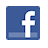Games
Problems
Go Pro!

# Set of Five Digit Numbers

Pro Problems > Math > Number and Quantity > Number Theory > Digits

## Set of Five Digit Numbers

S is the set of five-digit numbers such that the digits are in ascending order, there are no repeated digits, the sum of the first two digits is equal to the third digit, and the sum of the third and fourth digits is equal to the two more than the fifth digit. How many elements are in the set S? (Note that the leading digit cannot be a zero).

Presentation mode
Problem by Mr. Twitchell

## Solution

In order to make it feasible for teachers to use these problems in their classwork, no solutions are publicly visible, so students cannot simply look up the answers. If you would like to view the solutions to these problems, you must have a Virtual Classroom subscription.Assign this problem

## Similar Problems

### I Have Three Digits

I am a three digit number, and the following things are true about me:

1. The product of two of my digits is 8.
2. The sum of my digits is 13.
3. My first digit is four times my second digit.

What number am I?

### My Three Digits

I'm thinking of a three-digit number. The sum of my number's first and last digits is a perfect square. The sum of my number's first and second digits is also a perfect square. If my third digit is subtracted from my second digit, the result is 5. If my number is not a multiple of three, and it has no repeated digits, what is my number?

### Find the Number

My digits are all odd, and they add to 18. My first digit is four more than my last digit, the product of my digits is between 300 and 315, and I am less than 100,000. If my digits are not in descending order, what numbers could I be?

### Grapes on the Vine

The number of grapes on my grape vine is a three digit number. It is 7 times as much as the number of grapes on the vine last year, and 11 times the number of grapes on the vine the previous year. Next year, if I have twice as many grapes as I do this year, the number of grapes will still be a three digit number, but if I have three times as many grapes, the number of grapes will be a four digit number. If I have 21 times as many grapes, the number of grapes will be a five digit number.

If each jar of grape juice requires 20 grapes, how many full jars of grape juice can I make this year?

### Fiona's Telephone Number

When Shrek asks Fiona for her telephone number, Fiona is a bit coy about it, and tells Shrek the following information:

• My telephone number has 10 digits.
• There are no repeated digits in my telephone number.
• The first three digits are in ascending order.
• The second three digits are in descending order.
• Both the last four digits and the last two digits are multiples of sixty.
• My last four digits are not a multiple of 43.
• My first three digits are the square of an integer less than twenty.
• The sum of the second three digits is 14.

What number should Shrek dial?

### Two Digit Pattern Matching

How many two-digit numbers are there such that the digits match at least one of the following patterns:

1. The digits are both multiples of three.
2. Neither of the digits are multiples of two.
3. The digits add to 8.
4. The digits are perfect squares.

### Fill in the blanks

In the addition problem below, some digits are missing. They have been replaced by x and y. Find the values of x and y.

3xy2 + 3y1 = 40x3

### Three Digit Number

I'm thinking of a three-digit number. The sum of its digits is between 15 and 20 exclusive. The product of my first and last digits is 18. I don't have any repeated digits, and my digits are not in either ascending order or descending order. I am a multiple of three, but not of six. What number am I?

### Three Digits with Difference

I’m a three digit number, and the sum of my digits is 13. My first two digits differ by 3, and my last two digits differ by 5. What numbers could I be?

### Back to Back

X is a three-digit number. Y is the number obtained when the digits of X are reversed. Z is the six-digit number obtained by writing X and Y back to back, with X written first. W is the six-digit number obtained by writing Y and X back to back, with Y written first. What is the largest number which the sum of Z and W must be divisible by?

Happy New Year, Three Digit Difference, Four Digit Number, The Middle Palindrome, Palindrome Addition, Digits in a Multiplication Problem, Rhonda's Zip Code, Sum of Digits, Three Digits, sum and product, Coffee Math, Three Digit Number, All My Digits, Five Digit Number, Reverse Me# Featured Resources on This Site

Create Christmas ornaments and more
Print fun mazes in many different shapes

# Blogs on This SiteReviews and book lists - books we love!The site administrator fields questions from visitors.Like us on Facebook to get updates about new resources